# Continuous function facts for kids

Kids Encyclopedia Facts
(Redirected from Discontinuous)

A mathematical function is called continuous if, roughly said, a small change in the input only causes a small change in the output. If this is not the case, the function is discontinuous. Functions defined on the real numbers, with one input and one output variable, will show as an uninterrupted line (or curve). They can be drawn without lifting the pen off of the page. The definition given above was made by Augustin-Louis Cauchy.

Karl Weierstraß gave another definition of continuity: Imagine a function f, defined on the real numbers. At the point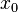$x_0$ the function will have the value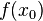$f(x_0)$. If the function is continuous at$x_0$, then for every value of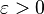$\varepsilon>0$ no matter how small it is, there is a value of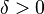$\delta > 0$, so that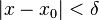$|x - x_0| < \delta$, means that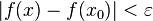$|f(x) - f(x_0)| < \varepsilon$. We can put this another way, given a point close to$x_0$ (called x), the absolute value of the difference between the two values of the function can be made increasingly small, if the point x is close enough to$x_0$.

There are also special forms of continuous, such as Lipschitz-continuous. A function is Lipschitz-continuous if there is a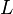$L$ with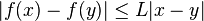$|f(x) - f(y)| \le L|x - y|$ for all x,y ∈ (a,b).